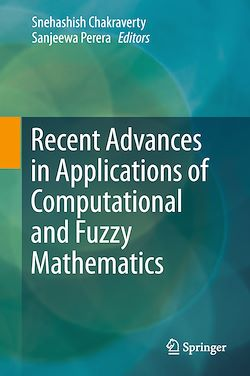178 Pages
English

# Recent Advances in Applications of Computational and Fuzzy Mathematics

-Description

This book addresses the basics of interval/fuzzy set theory, artificial neural networks (ANN) and computational methods. It presents step-by-step modeling for application problems along with simulation and numerical solutions.

In general, every science and engineering problem is inherently biased by uncertainty, and there is often a need to model, solve and interpret problems in the world of uncertainty. At the same time, exact information about models and parameters of practical applications is usually not known and precise values do not exist.

This book discusses uncertainty in both data and models. It consists of seven chapters covering various aspects of fuzzy uncertainty in application problems, such as shallow water wave equations, static structural problems, robotics, radon diffusion in soil, risk of invasive alien species and air quality quantification. These problems are handled by means of advanced computational and fuzzy theory along with machine intelligence when the uncertainties involved are fuzzy. The proposed computational methods offer new fuzzy computing methods that help other areas of knowledge construction where inexact information is present.

Subjects

##### IT systems

Informations